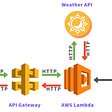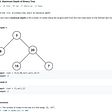# Leetcode 34: Find First and Last Position of Element in Sorted Array

`Input: nums = [5,7,7,8,8,10], target = 8Output: [3,4]`
`Input: nums = [5,7,7,8,8,10], target = 6Output: [-1,-1]`
`Input: nums = [], target = 0Output: [-1,-1]`
• `0 <= nums.length <= 105`
• `-109 <= nums[i] <= 109`
• `nums` is a non-decreasing array.
• `-109 <= target <= 109`
`class Solution {public:    vector<int> searchRange(vector<int>& nums, int target) {                  int start = 0;        int len = nums.size();        while (len > 0) {            int half = len >> 1;            int mid = start + half;            if (nums[mid] < target) {                start = mid + 1;                len = len - half - 1;            } else {                len = half;            }        }                if (start == nums.size() || nums[start] != target) return {-1, -1};                int end = 0;        len = nums.size() ;        while (len > 0) {            int half = len >> 1;            int mid = end + half;            if (nums[mid] > target) {                len = half;            } else {                end = mid + 1;                len = len - half - 1;            }        }                return {start, end - 1};    }};`

--

--

--

## More from dume0011

Love podcasts or audiobooks? Learn on the go with our new app.

## How to build HTTP REST APIs with AWS + Serverless Framework## How to Optimize the Hadoop cluster for high performance?## Why I failed at learning JavaScript and Python## The lazy way to manage Docker## I’m sick of lists of habits that are unrealistic for the majority of people.## The Spawn Mega Base is being built## 104 — Maximum Depth of a Binary Tree## LeetCode 1. Two Sum## Binary Tree Cameras